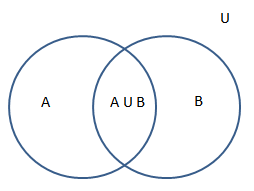Math Calculator

#### Union of Set | (A Union B) AUB Calculation

The union of two sets A and B is the set of elements which are in A, in B, or in both A and B. It is one of the set theories. Here is a simple online algebraic calculator that helps to find the union of two sets. Enter the value of set A and set B as shown and click calculate to obtain the union of two sets. (A union B) is represented as (AUB).

#### Calculator

 Enter the Set A Enter the Set B Union of sets A and B(AUB)

#### Example

if A = {1, 3, 5, 7} and B = {1, 2, 4, 6, 8} then (A ⋓ B) = {1, 2, 3, 4, 5, 6, 7, 8}.